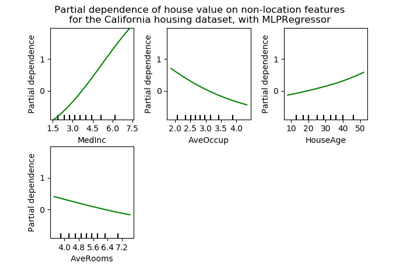# sklearn.inspection.partial_dependence¶

sklearn.inspection.partial_dependence(estimator, X, features, response_method=’auto’, percentiles=(0.05, 0.95), grid_resolution=100, method=’auto’)[source]

Partial dependence of features.

Partial dependence of a feature (or a set of features) corresponds to the average response of an estimator for each possible value of the feature.

Read more in the User Guide.

Parameters: estimator : BaseEstimator A fitted estimator object implementing predict, predict_proba, or decision_function. Multioutput-multiclass classifiers are not supported. X : array-like, shape (n_samples, n_features) X is used both to generate a grid of values for the features, and to compute the averaged predictions when method is ‘brute’. features : list or array-like of int The target features for which the partial dependency should be computed. response_method : ‘auto’, ‘predict_proba’ or ‘decision_function’, optional (default=’auto’) Specifies whether to use predict_proba or decision_function as the target response. For regressors this parameter is ignored and the response is always the output of predict. By default, predict_proba is tried first and we revert to decision_function if it doesn’t exist. If method is ‘recursion’, the response is always the output of decision_function. percentiles : tuple of float, optional (default=(0.05, 0.95)) The lower and upper percentile used to create the extreme values for the grid. Must be in [0, 1]. grid_resolution : int, optional (default=100) The number of equally spaced points on the grid, for each target feature. method : str, optional (default=’auto’) The method used to calculate the averaged predictions: ‘recursion’ is only supported for objects inheriting from BaseGradientBoosting, but is more efficient in terms of speed. With this method, X is only used to build the grid and the partial dependences are computed using the training data. This method does not account for the init predicor of the boosting process, which may lead to incorrect values (see warning below). With this method, the target response of a classifier is always the decision function, not the predicted probabilities. ‘brute’ is supported for any estimator, but is more computationally intensive. If ‘auto’, then ‘recursion’ will be used for BaseGradientBoosting estimators with init=None, and ‘brute’ for all other. averaged_predictions : ndarray, shape (n_outputs, len(values), len(values), …) The predictions for all the points in the grid, averaged over all samples in X (or over the training data if method is ‘recursion’). n_outputs corresponds to the number of classes in a multi-class setting, or to the number of tasks for multi-output regression. For classical regression and binary classification n_outputs==1. n_values_feature_j corresponds to the size values[j]. values : seq of 1d ndarrays The values with which the grid has been created. The generated grid is a cartesian product of the arrays in values. len(values) == len(features). The size of each array values[j] is either grid_resolution, or the number of unique values in X[:, j], whichever is smaller.

Warning

The ‘recursion’ method only works for gradient boosting estimators, and unlike the ‘brute’ method, it does not account for the init predictor of the boosting process. In practice this will produce the same values as ‘brute’ up to a constant offset in the target response, provided that init is a consant estimator (which is the default). However, as soon as init is not a constant estimator, the partial dependence values are incorrect for ‘recursion’.

Examples

>>> X = [[0, 0, 2], [1, 0, 0]]
>>> y = [0, 1]

## Examples using sklearn.inspection.partial_dependence¶